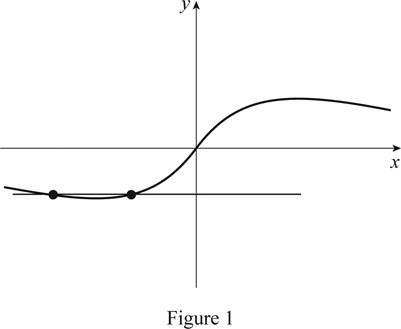# Whether the function given by a graph is one-to-one.### Single Variable Calculus: Concepts...

4th Edition
James Stewart
Publisher: Cengage Learning
ISBN: 9781337687805### Single Variable Calculus: Concepts...

4th Edition
James Stewart
Publisher: Cengage Learning
ISBN: 9781337687805

#### Solutions

Chapter 1.6, Problem 8E
To determine

## Whether the function given by a graph is one-to-one.

Expert Solution

The function is not a one-to one function.

### Explanation of Solution

Horizontal line test states that the graph of the function is one-to-one function if and only if a horizontal line intersects the graph exactly once.

Perform the horizontal line test for the given graph.

Draw a horizontal line such that it passes through the curve as shown in Figure 1.It is observed from Figure 1, the horizontal line intersects the curve at two distinct points, which means it fails the horizontal line test. Therefore, the function given by a graph is not a one-to-one function.

### Have a homework question?

Subscribe to bartleby learn! Ask subject matter experts 30 homework questions each month. Plus, you’ll have access to millions of step-by-step textbook answers!